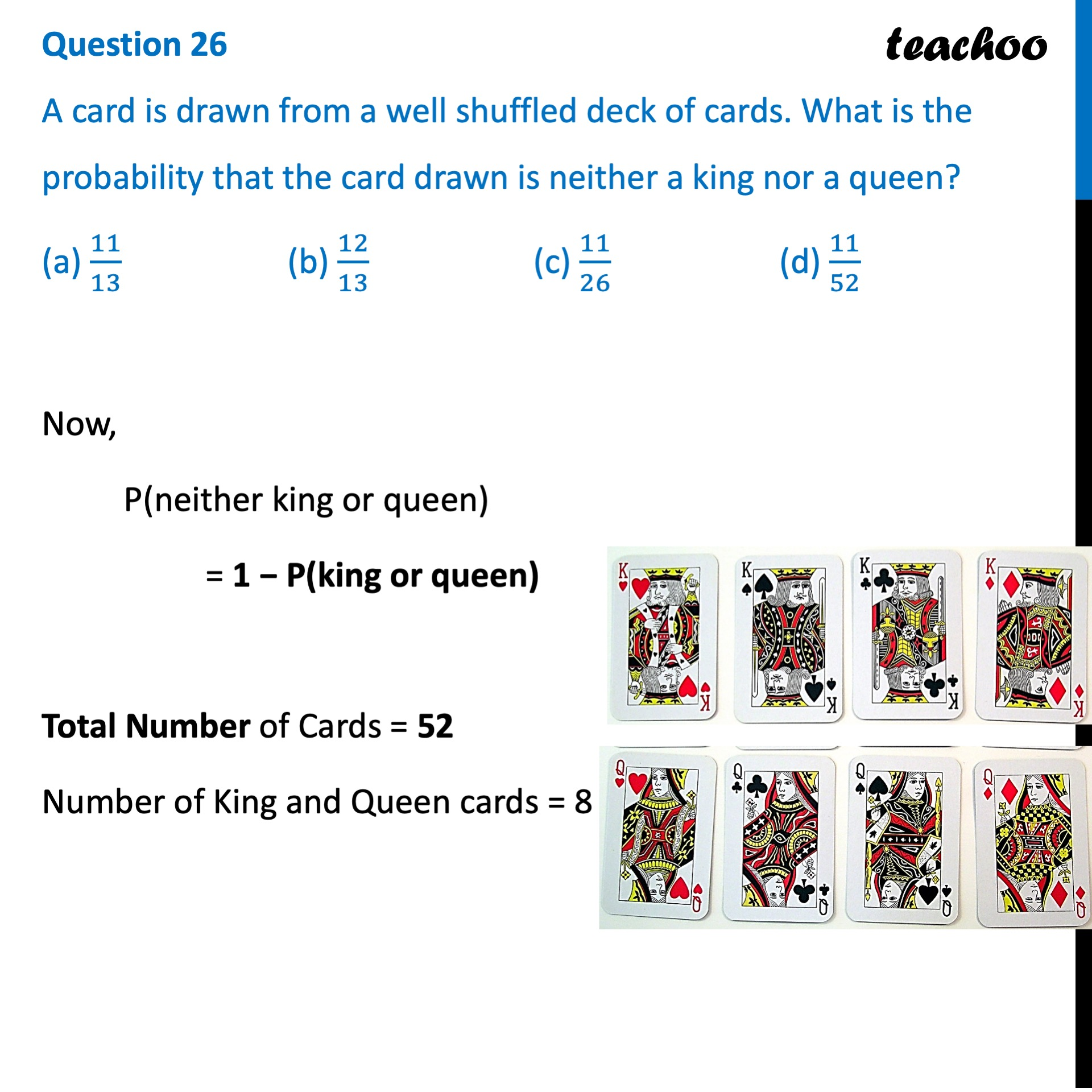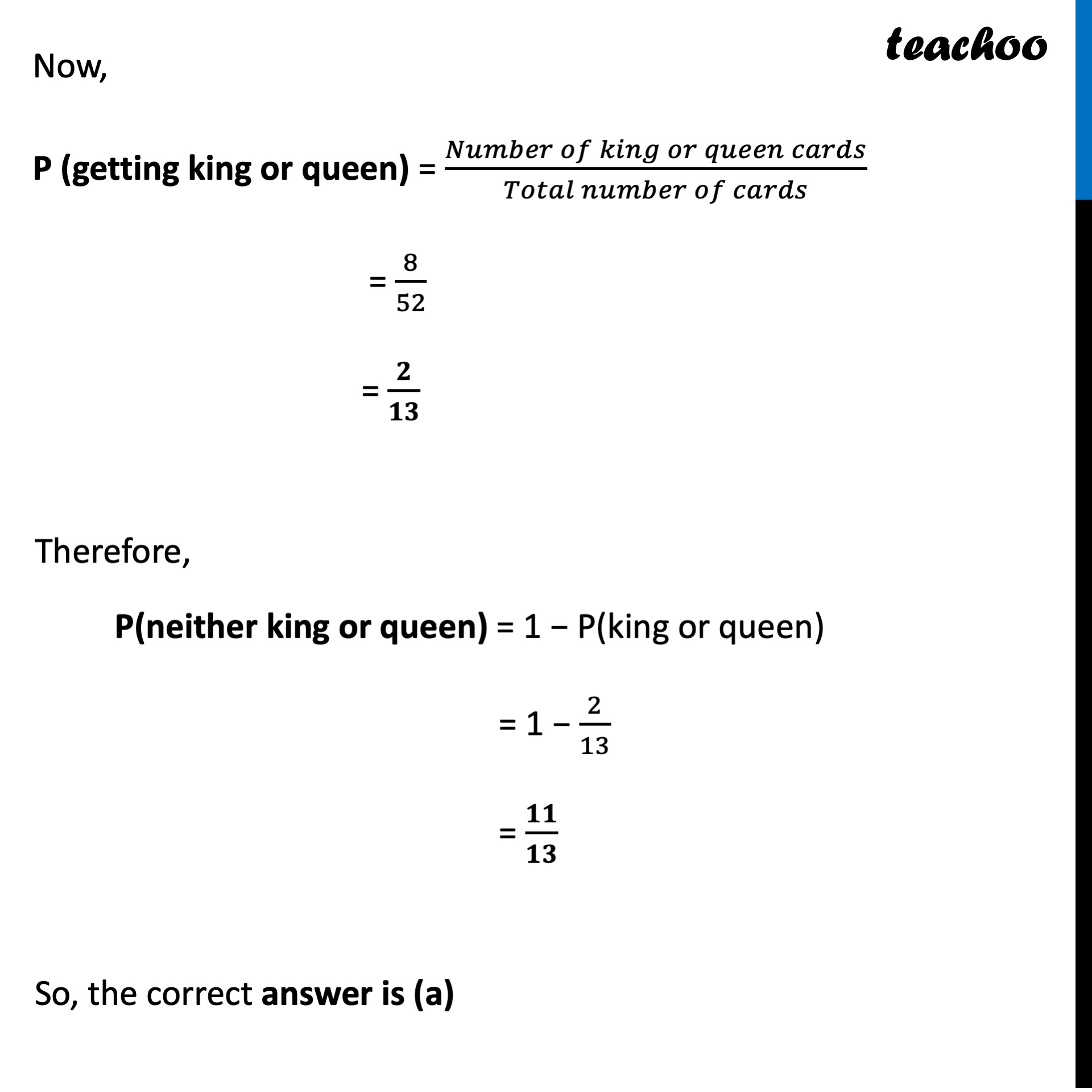## (a) 11/13  (b) 12/13   (c) 11/26   (d) 11/521. Class 10
2. Solutions of Sample Papers for Class 10 Boards
3. CBSE Class 10 Sample Paper for 2022 Boards - Maths Standard [MCQ]

Transcript

Question 26 A card is drawn from a well shuffled deck of cards. What is the probability that the card drawn is neither a king nor a queen? (a) 11/13 (b) 12/13 (c) 11/26 (d) 11/52 Now, P(neither king or queen) = 1 − P(king or queen) Total Number of Cards = 52 Number of King and Queen cards = 8 Now, P (getting king or queen) = (𝑁𝑢𝑚𝑏𝑒𝑟 𝑜𝑓 𝑘𝑖𝑛𝑔 𝑜𝑟 𝑞𝑢𝑒𝑒𝑛 𝑐𝑎𝑟𝑑𝑠)/(𝑇𝑜𝑡𝑎𝑙 𝑛𝑢𝑚𝑏𝑒𝑟 𝑜𝑓 𝑐𝑎𝑟𝑑𝑠) = 8/52 = 𝟐/𝟏𝟑 Therefore, P(neither king or queen) = 1 − P(king or queen) = 1 − 2/13 = 𝟏𝟏/𝟏𝟑 So, the correct answer is (a)